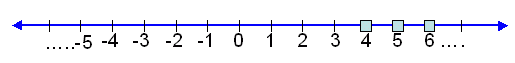Email us to get an instant 20% discount on highly effective K-12 Math & English kwizNET Programs!

#### Online Quiz (WorksheetABCD)

Questions Per Quiz = 2 4 6 8 10

### Middle/High School Algebra, Geometry, and Statistics (AGS)2.14 Representing Inequations on Number Line - I

 Example: Represent x > 3 on number line and x is a natural number. solution:If we take x = 0, we get 0 > 3. But 0 > 3 is not true. Therefore 0 is not a solution for the given inequation. If we take x = 1, we get 1 > 3. But 1 > 3 is not true. Therefore 1 is not a solution for the given inequation. If we take x = 2, we get 2 > 3. But 2 > 3 is not true. Therefore 2 is not a solution for the given inequation. If we take x = 3, we get 3 > 3. But 3 > 3 is not true. Therefore 3 is not a solution for the given inequation. If we take x = 4, we get 4 > 3. This is true. Therefore 4 is a solution for the given inequation. If we take x = 5, we get 5 > 3. This is true. Therefore 5 is a solution for the given inequation. Thus all the natural numbers greater than 3 are the solutions for the inequation x > 3. Directions: Draw the number line to the given inequalities. 1. Represent x > 2 on number line and x is a natural number. 2. Represent x > 5 on number line and x is a natural number. 3. Represent x > 4 on number line and x is a natural number. 4. Represent x > 6 on number line and x is a natural number. 5. Represent x > 8 on number line and x is a natural number.